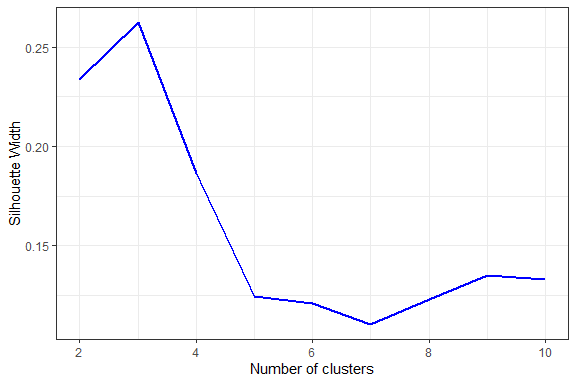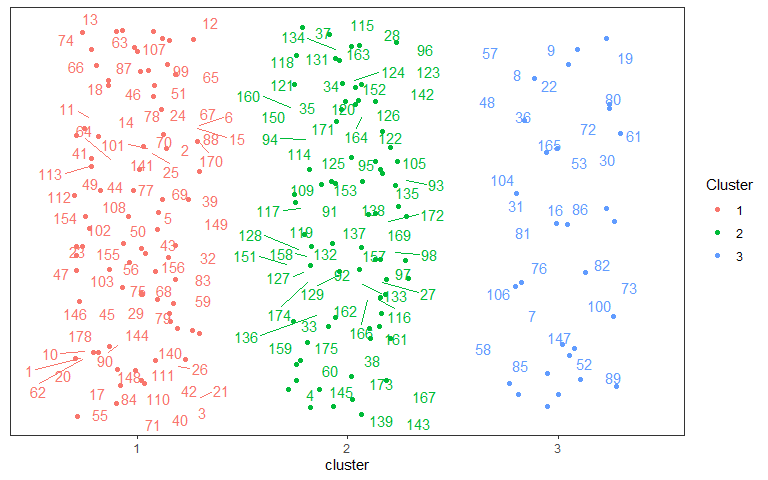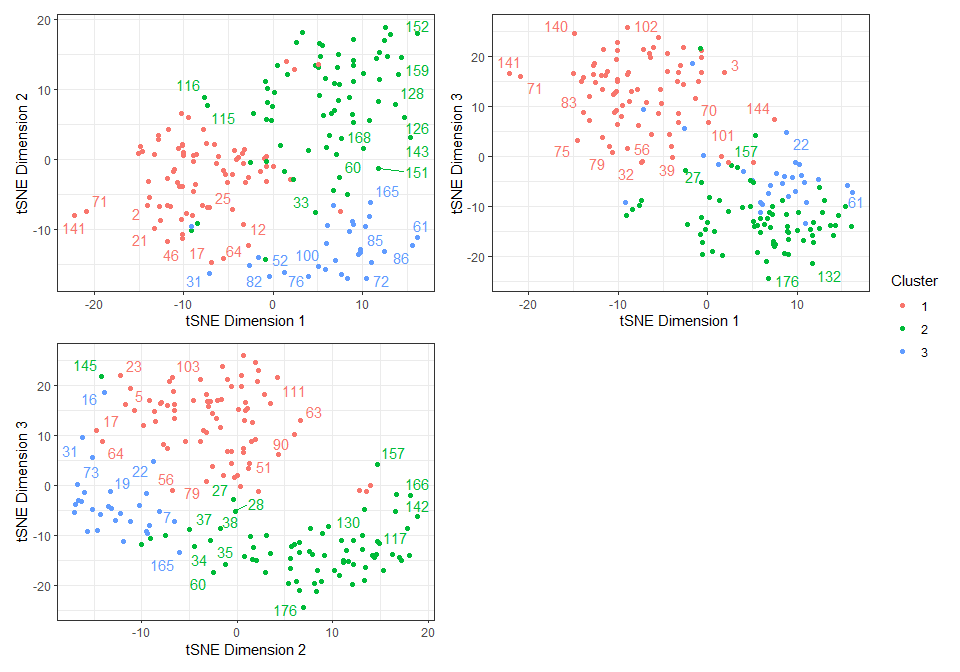# Character Partitioning

#### 2022-05-12

This vignette explains how to conduct automated morphological character partitioning as a pre-processing step for clock (time-calibrated) Bayesian phylogenetic analysis of morphological data, as introduced by .

## Installing package EvoPhylo

install.packages("EvoPhylo")
### OR
devtools::install_github("tiago-simoes/EvoPhylo")

## Character Partitioning

library(EvoPhylo)

### 1. Generate distance matrix

Generate a Gower distance matrix with get_gower_dist() by supplying the file path of a .nex file containing a character data matrix:

#Load a character data matrix and produce a Gower distance matrix
dist_matrix <- get_gower_dist("DataMatrix.nex", numeric = FALSE)

Below, we use the example data matrix characters that accompanies EvoPhylo.

data(characters)

dist_matrix <- get_gower_dist(characters, numeric = FALSE)

### 2. Estimate the optimal number of partitions

The optimal number of partitions (clusters) will be first determined using partitioning around medoids (PAM) with Silhouette widths index (Si) using get_sil_widths(). The latter will estimate the quality of each PAM cluster proposal relative to other potential clusters.

## Estimate and plot number of cluster against silhouette width
sw <- get_sil_widths(dist_matrix, max.k = 10)
plot(sw, color = "blue", size = 1)Decide on number of clusters based on plot; here, $$k = 3$$ partitions appears optimal.

### 3. Simple Workflow:

3.1. Analyze clusters with PAM under chosen $$k$$ value (from Si) with make_clusters().

3.2. Produce simple cluster graph

3.3. Export clusters/partitions to Nexus file with cluster_to_nexus().

## Generate and vizualize clusters with PAM under chosen k value.
clusters <- make_clusters(dist_matrix, k = 3)

plot(clusters)## Write clusters to Nexus file
cluster_to_nexus(clusters, file = "Clusters_Nexus.txt")

### 4. Complete Workflow:

4.1. Analyze clusters with PAM under chosen $$k$$ value (from Si) with make_clusters().

4.2. Produce a graphic clustering (tSNEs), coloring data points according to PAM clusters, to independently verify PAM clustering. This is set with the tsne argument within make_clusters().

4.3. Export clusters/partitions to Nexus file with cluster_to_nexus(). This can be copied and pasted into the Mr. Bayes command block.

#User may also generate clusters with PAM and produce a graphic clustering (tSNEs)
clusters <- make_clusters(dist_matrix, k = 3, tsne = TRUE, tsne_dim = 3)

plot(clusters, nrow = 2, max.overlaps = 5)#Write clusters/partitions in Nexus file format
cluster_to_nexus(clusters, file = "Clusters_Nexus.txt")